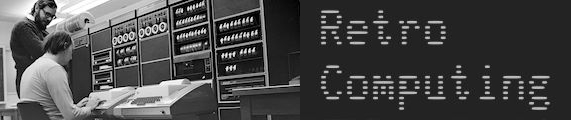# Speaking of Lunar Landers

Anyone fancy some typing in?From The Best of Creating Computer magazine Vol 1. Early 1970’s…

-Gordon

4 Likes

Hurrah! A bit of searching on key strings turned this up:
https://www.atariarchives.org/bcc1/showpage.php?page=265
and also
http://pdp-11.trailing-edge.com/rsts11/rsts-11-013/ROCKET.BAS

There are some quite groovy equations in there:

``````910 LET J=V+G*S+Z*(-Q-(Q^2)/2-(Q^3)/3-(Q^4)/4-(Q^5)/5)
920 LET I=A-G*S*S/2-V*S+Z*S*(Q/2+Q^2/6+Q^3/12+Q^4/20+Q^5/30)
``````

I see we have some accounting of fuel mass:

``620 LET M=M-S*K``
2 Likes

I’m rather fond of the the one in the August 1977 Byte. They actually build a model lander that is controlled by the computer.

Then there is the series that starts in the November 1977 issue on simulating motion, based on a lunar lander, from a NASA engineer.

Both of those start on page 18 of their respective issues.

2 Likes

Much clearer (and OCRd) scan of the Creative Computing article here:

1 Like